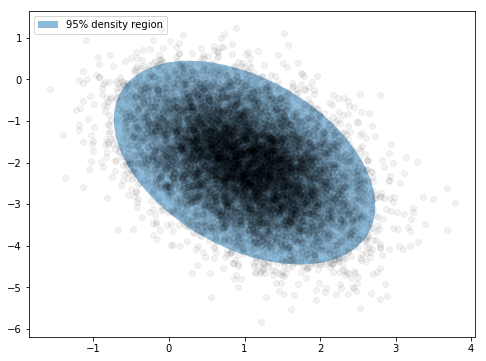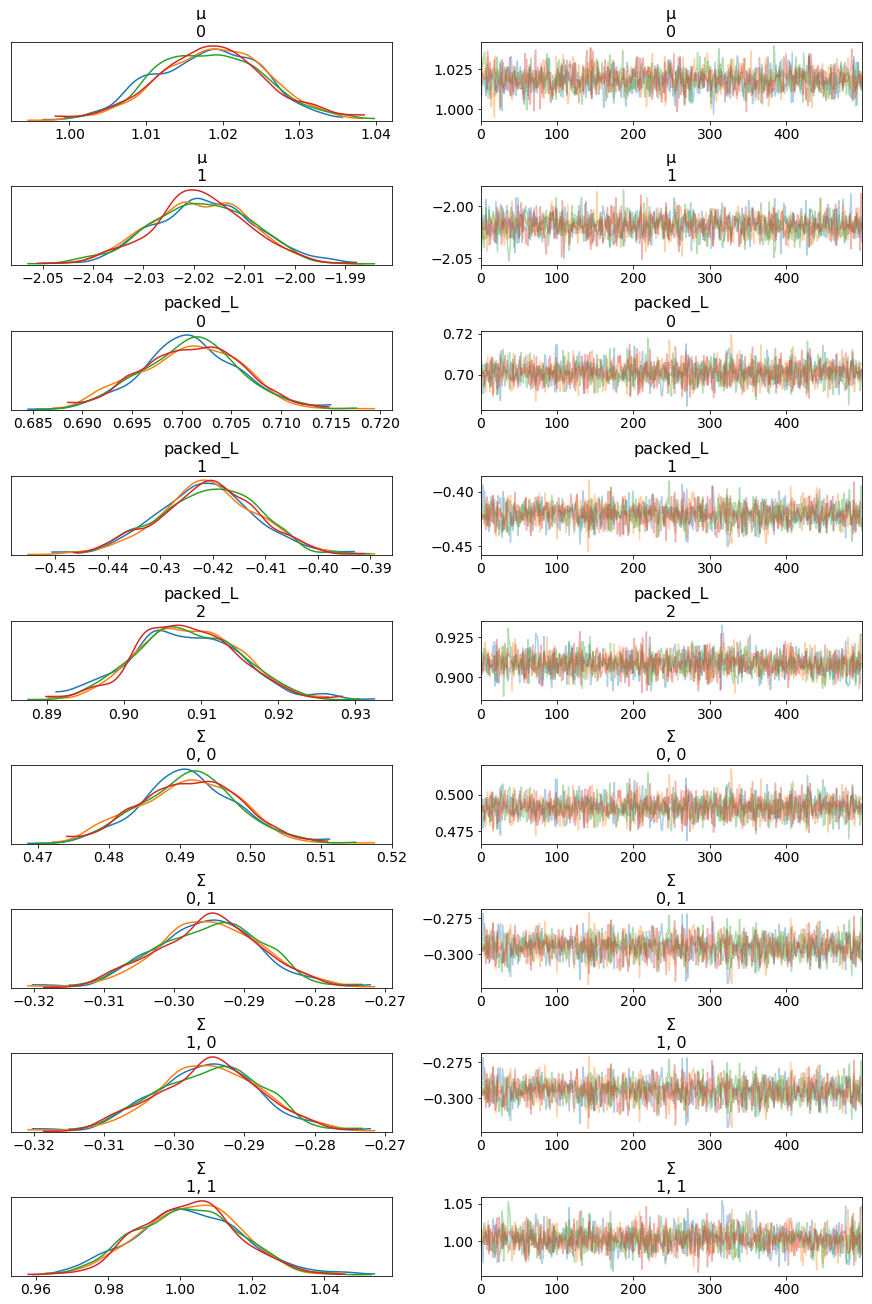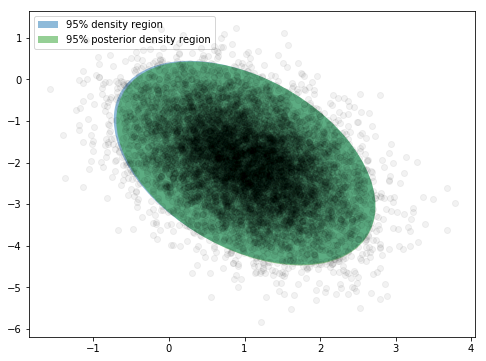# LKJ Cholesky Covariance Priors for Multivariate Normal Models¶

While the inverse-Wishart distribution is the conjugate prior for the covariance matrix of a multivariate normal distribution, it is not very well-suited to modern Bayesian computational methods. For this reason, the LKJ prior is recommended when modeling the covariance matrix of a multivariate normal distribution.

To illustrate modelling covariance with the LKJ distribution, we first generate a two-dimensional normally-distributed sample data set.

In :
%matplotlib inline

In :
from warnings import filterwarnings

In :
from matplotlib.patches import Ellipse
from matplotlib import pyplot as plt
import numpy as np
import pymc3 as pm
import seaborn as sns

In :
filterwarnings('ignore', message='findfont')

In :
SEED = 3264602 # from random.org

np.random.seed(SEED)

In :
N = 10000

μ_actual = np.array([1, -2])
Σ_actual = np.array([[0.5, -0.3],
[-0.3, 1.]])

x = np.random.multivariate_normal(μ_actual, Σ_actual, size=N)

In :
var, U = np.linalg.eig(Σ_actual)
angle = 180. / np.pi * np.arccos(np.abs(U[0, 0]))

In :
fig, ax = plt.subplots(figsize=(8, 6))

blue, _, red, *_ = sns.color_palette()

e = Ellipse(μ_actual, 2 * np.sqrt(5.991 * var),
2 * np.sqrt(5.991 * var),
angle=angle)
e.set_alpha(0.5)
e.set_facecolor(blue)
e.set_zorder(10);

ax.scatter(x[:, 0], x[:, 1], c='k', alpha=0.05, zorder=11);

rect = plt.Rectangle((0, 0), 1, 1, fc=blue, alpha=0.5)
ax.legend([rect], ['95% density region'], loc=2);The sampling distribution for the multivariate normal model is $\mathbf{x} \sim N(\mu, \Sigma)$, where $\Sigma$ is the covariance matrix of the sampling distribution, with $\Sigma_{ij} = \textrm{Cov}(x_i, x_j)$. The density of this distribution is

$$f(\mathbf{x}\ |\ \mu, \Sigma^{-1}) = (2 \pi)^{-\frac{k}{2}} |\Sigma|^{-\frac{1}{2}} \exp\left(-\frac{1}{2} (\mathbf{x} - \mu)^{\top} \Sigma^{-1} (\mathbf{x} - \mu)\right).$$

The LKJ distribution provides a prior on the correlation matrix, $\mathbf{C} = \textrm{Corr}(x_i, x_j)$, which, combined with priors on the standard deviations of each component, induces a prior on the covariance matrix, $\Sigma$. Since inverting $\Sigma$ is numerically unstable and inefficient, it is computationally advantageous to use the Cholesky decompositon of $\Sigma$, $\Sigma = \mathbf{L} \mathbf{L}^{\top}$, where $\mathbf{L}$ is a lower-triangular matrix. This decompositon allows computation of the term $(\mathbf{x} - \mu)^{\top} \Sigma^{-1} (\mathbf{x} - \mu)$ using back-substitution, which is more numerically stable and efficient than direct matrix inversion.

PyMC3 supports LKJ priors for the Cholesky decomposition of the covariance matrix via the LKJCholeskyCov distribution. This distribution has parameters n and sd_dist, which are the dimension of the observations, $\mathbf{x}$, and the PyMC3 distribution of the component standard deviations, repsectively. It also has a hyperparamter eta, which controls the amount of correlation between components of $\mathbf{x}$. The LKJ distribution has the density $f(\mathbf{C}\ |\ \eta) \propto |\mathbf{C}|^{\eta - 1}$, so $\eta = 1$ leads to a uniform distribution on correlation matrices, while the magnitude of correlations between components decreases as $\eta \to \infty$.

In this example, we model the standard deviations with $\textrm{HalfCauchy}(2.5)$ priors, and the correlation matrix as $\mathbf{C} \sim \textrm{LKJ}(\eta = 2)$.

In :
with pm.Model() as model:
packed_L = pm.LKJCholeskyCov('packed_L', n=2,
eta=2., sd_dist=pm.HalfCauchy.dist(2.5))


Since the Cholesky decompositon of $\Sigma$ is lower triangular, LKJCholeskyCov only stores the diagonal and sub-diagonal entries, for efficiency.

In :
packed_L.tag.test_value.shape

Out:
(3,)

We use expand_packed_triangular to transform this vector into the lower triangular matrix $\mathbf{L}$, which appears in the Cholesky decomposition $\Sigma = \mathbf{L} \mathbf{L}^{\top}$.

In :
with model:
L = pm.expand_packed_triangular(2, packed_L)
Σ = pm.Deterministic('Σ', L.dot(L.T))

/Users/twiecki/anaconda3/lib/python3.6/site-packages/theano/tensor/subtensor.py:2197: FutureWarning: Using a non-tuple sequence for multidimensional indexing is deprecated; use arr[tuple(seq)] instead of arr[seq]. In the future this will be interpreted as an array index, arr[np.array(seq)], which will result either in an error or a different result.
rval = inputs.__getitem__(inputs[1:])
/Users/twiecki/anaconda3/lib/python3.6/site-packages/theano/tensor/subtensor.py:2339: FutureWarning: Using a non-tuple sequence for multidimensional indexing is deprecated; use arr[tuple(seq)] instead of arr[seq]. In the future this will be interpreted as an array index, arr[np.array(seq)], which will result either in an error or a different result.
out[inputs[2:]] = inputs

In :
L.tag.test_value.shape

Out:
(2, 2)

To complete our model specification, we place independent, vague normal priors, $\mu_i \sim N(0, 10^2),$ on the components of $\mu$.

In :
with model:
μ = pm.Normal('μ', 0., 10., shape=2,
testval=x.mean(axis=0))
obs = pm.MvNormal('obs', μ, chol=L, observed=x)


We sample from this model using NUTS.

In [ ]:
with model:
trace = pm.sample(random_seed=SEED, cores=4)


Both the traces and Gelman-Rubin statistics indicate excellent convergence.

In :
pm.traceplot(trace);

/Users/twiecki/anaconda3/lib/python3.6/site-packages/theano/tensor/subtensor.py:2339: FutureWarning: Using a non-tuple sequence for multidimensional indexing is deprecated; use arr[tuple(seq)] instead of arr[seq]. In the future this will be interpreted as an array index, arr[np.array(seq)], which will result either in an error or a different result.
out[inputs[2:]] = inputsIn :
max(np.max(gr_stats) for gr_stats in pm.gelman_rubin(trace).values())

Out:
1.0006858

We see that the posterior expected value of the each component of $\mu$ and $\Sigma$ is within 2% of the true value.

In :
μ_post = trace['μ'].mean(axis=0)
1 - μ_post / μ_actual

Out:
array([-0.01835096, -0.00930619])
In :
Σ_post = trace['Σ'].mean(axis=0)
1 - Σ_post / Σ_actual

Out:
array([[ 0.01736307,  0.01615312],
[ 0.01615312, -0.00225496]])

The following plot also shows excellent visual agreement between the true distribution and the posterior distribution.

In :
var_post, U_post = np.linalg.eig(Σ_post)
angle_post = 180. / np.pi * np.arccos(np.abs(U_post[0, 0]))

In :
fig, ax = plt.subplots(figsize=(8, 6))

e = Ellipse(μ_actual, 2 * np.sqrt(5.991 * var),
2 * np.sqrt(5.991 * var),
angle=angle)
e.set_alpha(0.5)
e.set_facecolor(blue)
e.set_zorder(10);

e_post = Ellipse(μ_post, 2 * np.sqrt(5.991 * var_post),
2 * np.sqrt(5.991 * var_post),
angle=angle_post)
e_post.set_alpha(0.5)
e_post.set_facecolor(red)
e_post.set_zorder(10);